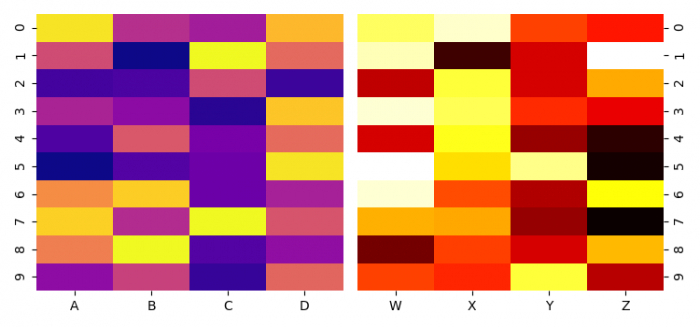# Combining two heatmaps in seaborn

To combine two heatmaps in seaborn, we can take the following steps −

## Steps

• Set the figure size and adjust the padding between and around the subplots.

• Create two Pandas data frames.

• Create a figure and a set of subplots, ax1 and ax2.

• Plot the rectangular data as a color-encoded matrix, on ax1 and ax2.

• Move ticks and ticklabels (if present) to the right of the axes.

• Keep the width of the padding between subplots minimum, as a fraction of the average axes width.

• To display the figure, use show() method.

## Example

import matplotlib.pyplot as plt
import numpy as np
import pandas as pd
import seaborn as sns

plt.rcParams["figure.figsize"] = [7.50, 3.50]
plt.rcParams["figure.autolayout"] = True

df1 = pd.DataFrame(np.random.rand(10, 4), columns=list("ABCD"))
df2 = pd.DataFrame(np.random.rand(10, 4), columns=list("WXYZ"))

fig, (ax1, ax2) = plt.subplots(ncols=2)

sns.heatmap(df1, cmap="plasma", ax=ax1, cbar=False)
sns.heatmap(df2, cmap="hot", ax=ax2, cbar=False)

ax2.yaxis.tick_right()

plt.show()

## Output

It will produce the following output −Updated on: 02-Feb-2022

5K+ Views